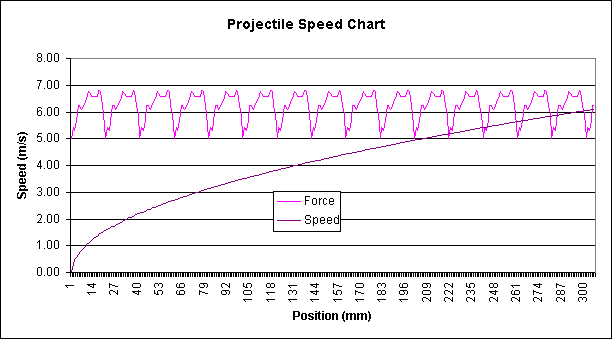# Computing Coil Timing for Mark I

In spreadsheet #1 and spreadsheet #2 we took measurements and calculated the forces of acceleration. Here, we go further to derive and compute the equations of motion (time, speed, distance) for the projectile.

These types of equations were once solved on special Navy ballistics computers -- originally using analog computers! Now you can solve the same thing in a fraction of a second of computation on your home computer. You get nicer graphs, too.

What have we got so far? We know the acceleration of the projectile at each mm position. That's important. From that, we can derive its new speed after it travels that 1mm distance. From the speed we can derive the exact time it takes the projectile to move 1mm. This tells us when the projectile will be where. Then we can choose when to turn each coil on and off.

Why don't we just turn on each coil for the same amount of time? Because that yields a travelling magnetic wave that moves at a constant velocity. (Assuming each coil is the same length.) If it launches anything at all, the first coil will bring it up to that speed, and all subsequent coils will simply maintain that same velocity. No, we need a different timing for each coil. The projectile spends a long time in coil #1 as it starts moving, a shorter time in coil #2 as it moves faster, and it spends a really short amount of time in the last coil!

## Equations of Motion

Remember your high school physics? (Have you been through high school?) Let's trot out those basic equations of motion, and use them to fill in a spreadsheet.

The first basic fact, which we'll take as a given, tells us the new velocity as a function of acceleration and time. Under a constant acceleration ai, with current velocity vi, and a small increment of time dt, the new velocity vi+1 is:

vi+1 = vi + ai dt

The second basic fact tells us the new position (distance) as a function of velocity and time. The new distance d after an increment in time dt at constant velocity v works like this:

di+1 = di + vi dt

We know the distance between measurements is 1mm, but not the time at each 1mm interval. So we solve the above equations backwards for the time dt, and you get:

dti+1 = (SQRT(vi*vi+2*ai*d)-vi)/ai

And that is the formula that goes into each time cell of the spreadsheet.

## Projectile Speed Chart

Let's plot a speed chart. But first, here's a few design choices I made.

In my design, I chose to use only the middle half of the acceleration profile. That is, I have two coils active at all times. The coils are short, but electrically they look twice as long as one coil. The projectile is always partway into a coil when it turns on, and I completely avoid the timing-critical sections of low acceleration as the projectile coasts out of one coil before it reaches the next. The change in speed is the area under the acceleration curve, so I chose to use the highest portion of the acceleration profile.

Here's the speed chart from the spreadsheet. It assumes there's 16 coils just to see what would happen. I'm using hand-wound coils; I would never wind that many because both hands would fall off! The chart displays both acceleration and speed on the same graph. You can see the middle portion of the acceleration profile is repeated for each coil along the gun.My goal was 5.8 m/s. This chart shows it would take 15 coils of this strength to achieve that speed. I think I'll just build only the first three or four coils, and see what happens. The chart shows I should get about half the desired speed of the goal with a quarter of the work. It would be a lot easier to create a larger power supply than to build all 16 coils, drivers and timing logic.

You can see the biggest boost is given by the first few coils of the gun. It seems to me there is little benefit in ever building more than about four coils. Gee, I really wonder if the kilometer-long mass driver project realizes what a diminishing benefit comes from the last half of the drivers!?